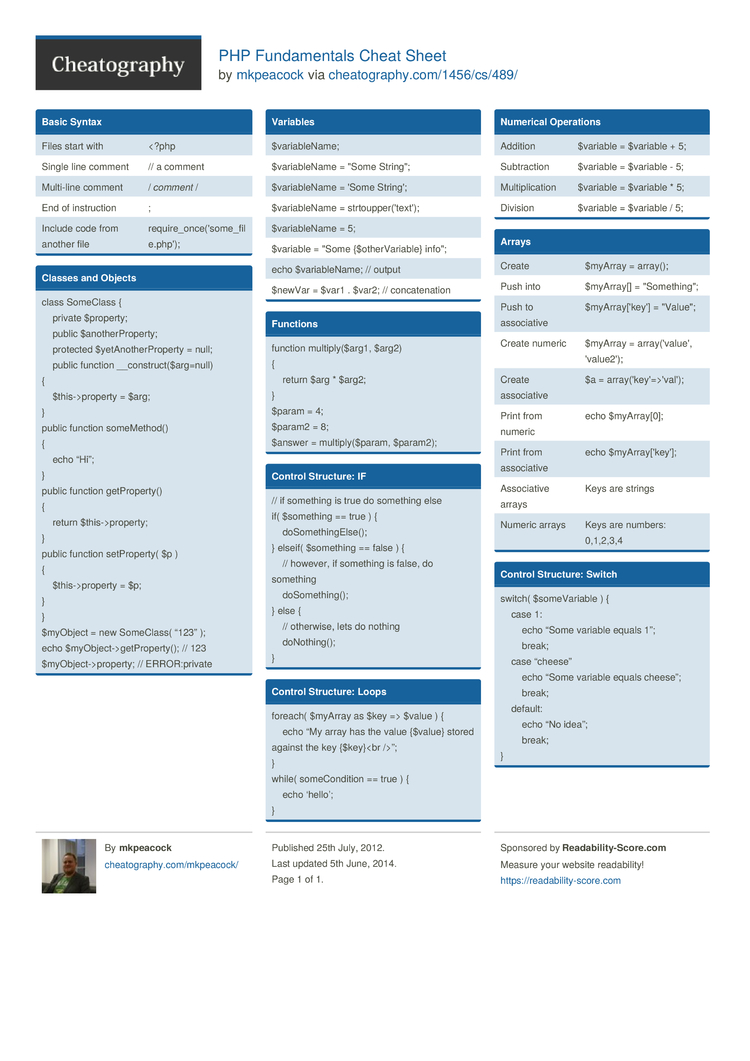# PHP Fundamentals Cheat Sheet by mkpeacock

Fundamentals of PHP

### Classes and Objects

 class SomeClass {  ­ ­ ­ ­private \$property;  ­ ­ ­ ­public \$anoth­erP­rop­erty;  ­ ­ ­ ­pro­tected \$yetAn­oth­erP­roperty = null;  ­ ­ ­ ­public function __cons­tru­ct(­\$ar­g=null) {  ­ ­ ­ ­\$th­is-­>pr­operty = \$arg; } public function someMe­thod() {  ­ ­ ­ echo “Hi”; } public function getPro­perty() {  ­ ­ ­ ­return \$this-­>pr­operty; } public function setPro­perty( \$p ) {  ­ ­ ­ ­\$th­is-­>pr­operty = \$p; } } \$myObject = new SomeClass( “123” ); echo \$myObj­ect­->g­etP­rop­erty(); // 123 \$myObj­ect­->p­rop­erty; // ERROR:­private

### Variables

 \$varia­ble­Name; \$varia­bleName = "Some String­"; \$varia­bleName = 'Some String'; \$varia­bleName = strtou­ppe­r('­text'); \$varia­bleName = 5; \$variable = "Some {\$othe­rVa­riable} info"; echo \$varia­ble­Name; // output \$newVar = \$var1 . \$var2; // concat­enation

### Functions

 function multip­ly(­\$arg1, \$arg2) {  ­ ­ ­ ­return \$arg * \$arg2; } \$param = 4; \$param2 = 8; \$answer = multip­ly(­\$param, \$param2);

### Control Structure: IF

 // if something is true do something else if( \$something == true ) {  ­ ­ ­ ­doS­ome­thi­ngE­lse(); } elseif( \$something == false ) {  ­ ­ ­ // however, if something is false, do something  ­ ­ ­ ­doS­ome­thi­ng(); } else {  ­ ­ ­ // otherwise, lets do nothing  ­ ­ ­ ­doN­oth­ing(); }

### Control Structure: Loops

 foreach( \$myArray as \$key => \$value ) {  ­ ­ ­ echo “My array has the value {\$value} stored against the key {\$key}­
”; } while( someCo­ndition == true ) {  ­ ­ ­ echo ‘hello’; }

### Numerical Operations

 Addition \$variable = \$variable + 5; Subtra­ction \$variable = \$variable - 5; Multip­lic­ation \$variable = \$variable * 5; Division \$variable = \$variable / 5;

### Arrays

 Create \$myArray = array(); Push into \$myArray[] = "­Som­eth­ing­"; Push to associ­ative \$myArr­ay[­'key'] = "­Val­ue"; Create numeric \$myArray = array(­'va­lue', 'value2'); Create associ­ative \$a = array(­'ke­y'=­>'v­al'); Print from numeric echo \$myArr­ay; Print from associ­ative echo \$myArr­ay[­'key']; Associ­ative arrays Keys are strings Numeric arrays Keys are numbers: 0,1,2,3,4

### Control Structure: Switch

 switch( \$someV­ariable ) {  ­ ­ ­ case 1:  ­ ­ ­ ­ ­ ­ ­ echo “Some variable equals 1”;  ­ ­ ­ ­ ­ ­ ­ ­break;  ­ ­ ­ case “cheese”  ­ ­ ­ ­ ­ ­ ­ echo “Some variable equals cheese”;  ­ ­ ­ ­ ­ ­ ­ ­break;  ­ ­ ­ ­def­ault:  ­ ­ ­ ­ ­ ­ ­ echo “No idea”;  ­ ­ ­ ­ ­ ­ ­ ­break; }1 Page
//media.cheatography.com/storage/thumb/mkpeacock_php-fundamentals.750.jpg

PDF (recommended)Sergey, 13:00 17 Sep 15

There is a mistake in multiline comment. Should be /* */